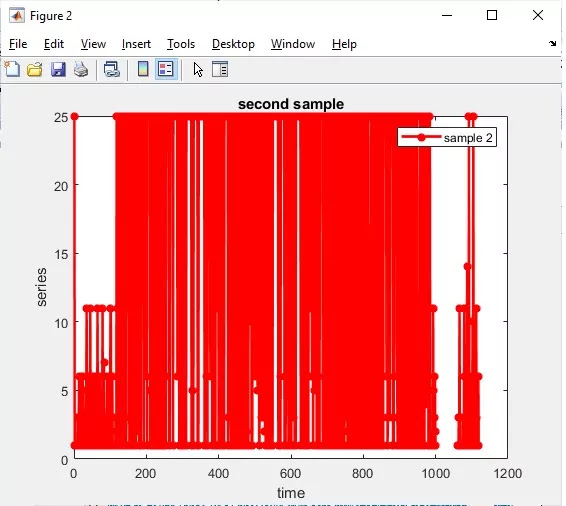How to easily convert .csv file to .mat file in MATLAB - Matlab Simulink for E & T

## Tuesday, August 4, 2020How to convert .csv file to .mat file in MATLAB

# Easily Convert .Csv File To .Mat File in Matlab and Plot the Values in a Graph

• Initially convert the .csv file (used a .csv file with three numeric variables and with 1000+ entries) to table by importing it in MATLAB. After importing if you need to generate a script means you can follow the steps in the video.
• Then since we have created table file we have to convert it into .mat file for that using table2array default function
• Next  plotted these results in a line graph.

For most suiatable laptop for Matlab-  https://amzn.to/39QeZ9d

Camera- https://amzn.to/39KBi02

professional tripod- https://amzn.to/39Jg96w

Please don't forget to Like, Share, Comment and Subscribe to Channel for more videos.

For most suiatable laptop for Matlab-  https://amzn.to/39QeZ9d

Camera- https://amzn.to/39KBi02

professional tripod- https://amzn.to/39Jg96w

Full Matlab Code

%% Import data from text file

% Script for importing data from the following text file:

%

%

%% Setup the Import Options

opts = delimitedTextImportOptions('NumVariables', 3);

% Specify range and delimiter

opts.DataLines = [1, Inf];

opts.Delimiter = ',';

% Specify column names and types

opts.VariableNames = ['VarName1', 'VarName2', 'VarName3'];

opts.VariableTypes = ['double', 'double', 'double'];

opts.ExtraColumnsRule = 'ignore';

% Import the data

sampledata = readtable('F:\sample code\myworks\matlab\text mining\plot csv data\sample_data.csv', opts);

%% Clear temporary variables

clear opts

sampledata=table2array(sampledata);

%%plot these variables in graph

figure;

plot(sampledata(:,1))

legend('sample 1')

xlabel('time')

ylabel('series')

title('first sample')

grid on

pause(0.5)

% Plot results

figure;

plot(sampledata(:,2),'r*-','linewidth',2)

legend('sample 2')

xlabel('time')

ylabel('series')

title('second sample')

pause(0.5)

% Plot results

figure;

plot(sampledata(:,3),'r*-','linewidth',2)

legend('sample 3')

xlabel('time')

ylabel('series')

title('third sample')

grid on

## Matlab Output Result

For most suiatable laptop for Matlab-  https://amzn.to/39QeZ9d

Camera- https://amzn.to/39KBi02

professional tripod- https://amzn.to/39Jg96w

Results Line graphs for three variables

first variable as sample 1 second as sample 2 and third as sample 3.For most suiatable laptop for Matlab-  https://amzn.to/39QeZ9d

Camera- https://amzn.to/39KBi02

professional tripod- https://amzn.to/39Jg96wFor most suiatable laptop for Matlab-  https://amzn.to/39QeZ9d

Camera- https://amzn.to/39KBi02

professional tripod- https://amzn.to/39Jg96w